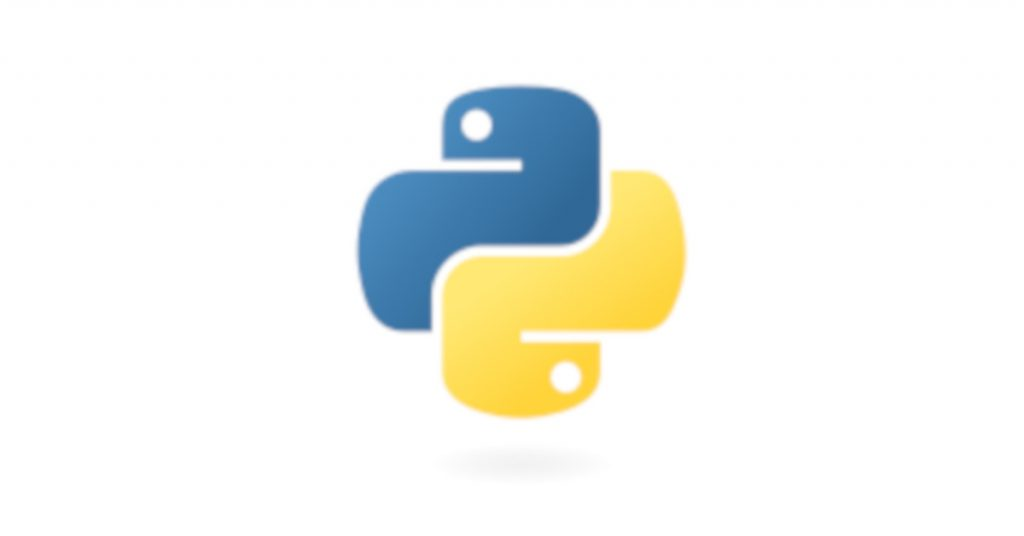# How to Convert a CSV to NumPy Array in Python?

## Problem Formulation

Given a CSV file (e.g., stored in the file with name `'my_file.csv'`).

````INPUT: file 'my_file.csv'`
```9,8,7
6,5,4
3,2,1``````

Challenge: How to convert it to a NumPy Array?

````OUTPUT: 2D NumPy Array`
```[[9. 8. 7.]
[6. 5. 4.]
[3. 2. 1.]]``````You can convert a CSV file to a NumPy array simply by calling `np.loadtxt()` with two arguments: the `filename` and the `delimiter` string. For example, the expression `np.loadtxt('my_file.csv', delimiter=',')` returns a NumPy array from the `'my_file.csv'` with delimiter symbols `','`.

Here’s an example:

```import numpy as np

print(array)```

Output:

``````[[9. 8. 7.]
[6. 5. 4.]
[3. 2. 1.]]``````You can convert a CSV file with first-line header to a NumPy array by calling `np.loadtxt()` with three arguments: the `filename`, `skiprows=1` to skip the first line (header), and the `delimiter` string. For example, the expression `np.loadtxt('my_file.csv', skiprows=1, delimiter=',')` returns a NumPy array from the `'my_file.csv'` with delimiter symbols `','` while skipping the first line.

Here’s an example:

```import numpy as np

print(array)```

Output:

``````[[9. 8. 7.]
[6. 5. 4.]
[3. 2. 1.]]``````To convert a CSV file `'my_file.csv'` into a list of lists in Python, use the `csv.reader(file_obj)` method to create a CSV file reader. Then convert the resulting object to a list using the `list()` constructor. As a final step, you can convert the nested list to a NumPy array by using the `np.array(list)` constructor.

Here’s an example:

```import numpy as np
import csv

csv_filename = 'my_file.csv'

with open(csv_filename) as f:
print(lst)```

The output is the list of lists:

``[['9', '8', '7'], ['6', '5', '4'], ['3', '2', '1']]``

Now, if you need to convert it to a NumPy array, you can simply use the `np.array()` function on the newly-created list like so:

```array = np.array(lst)
print(array)```

Output:

``````[['9' '8' '7']
['6' '5' '4']
['3' '2' '1']]``````

🌎 Related Tutorial: How to Convert CSV to List of Lists in Python

## Method 4: np.genfromtxt()np.genfromtxt()

You can convert a CSV file to a NumPy array simply by calling `np.genfromtxt()` with two arguments: the `filename` and the `delimiter` string. For example, the expression `np.genfromtxt('my_file.csv', delimiter=',')` returns a NumPy array from the `'my_file.csv'` with delimiter symbol `','`.

Here’s an example:

```import numpy as np

print(array)```

Output:

``````[[9. 8. 7.]
[6. 5. 4.]
[3. 2. 1.]]``````

## Method 5: Pandas read_csv() and df.to_numpy()A quick and efficient way to read a CSV to a NumPy array is to combine Pandas’ `pd.read_csv()` function to read a given CSV file to a DataFrame with the `df.to_numpy()` function to convert the Pandas DataFrame to a NumPy array.

Here’s an example:

```import pandas as pd

array = df.to_numpy()

print(array)
```

Output:

``````[[9 8 7]
[6 5 4]
[3 2 1]]``````

🌎 Related Tutorial: 17 Ways to Read a CSV File to a Pandas DataFrame

## Summary

We have seen five ways to convert a CSV file to a 2D NumPy array:

• Method 1: `np.loadtxt()`
• Method 2: `np.loadtxt()` with Header
• Method 4: `np.genfromtxt()`
• Method 5: Pandas `read_csv()` and `df.to_numpy()`
Our preferred way is `np.loadtxt()` for its simplicity and Pandas for its extensibility.